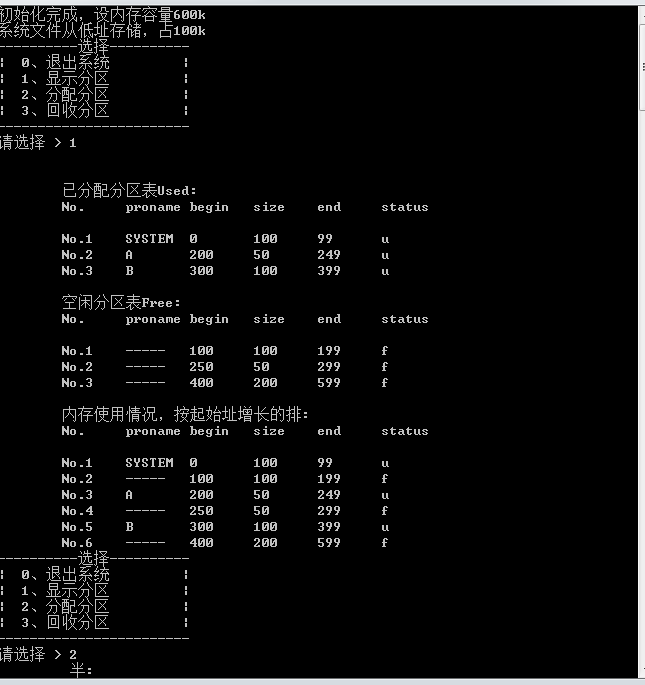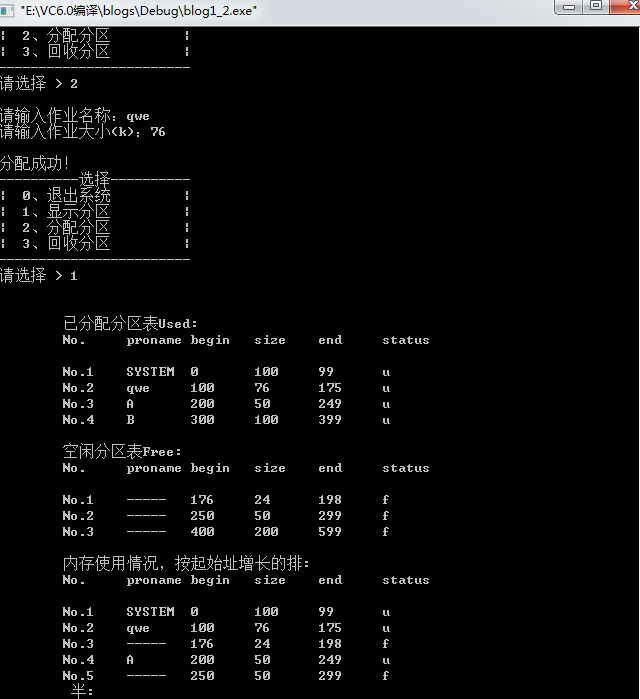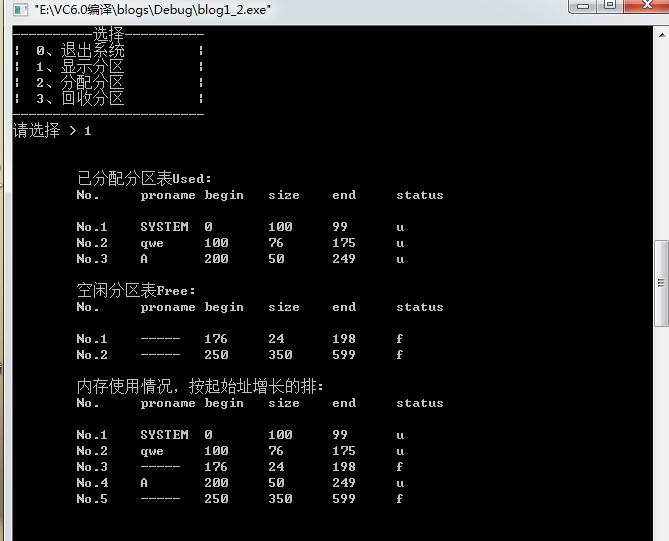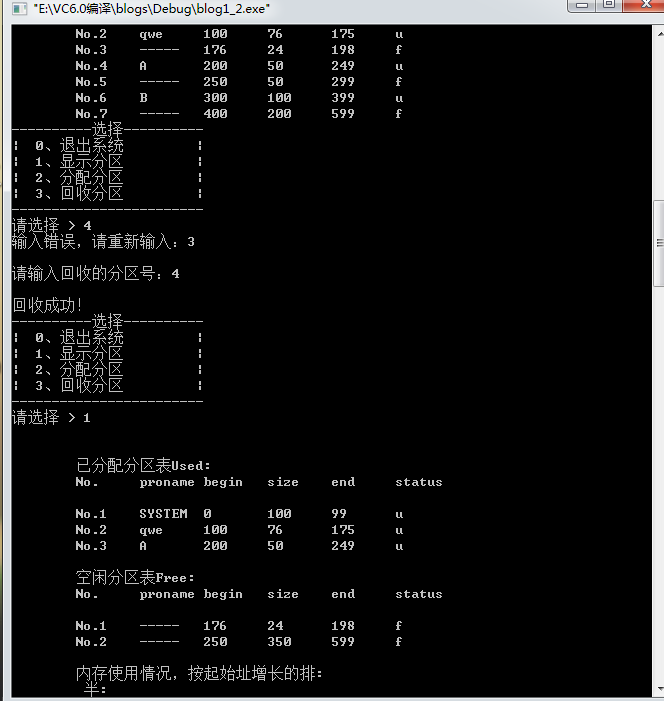• 内存分配算法的实现实验报告
千次阅读
2021-05-21 13:12:12

动态分区分配算法实验报告(共10篇)

动态分区分配算法实验报告(共10篇) 实验四动态分区分配算法实验报告及程序

实验报告四 动态分区分配算法

班级 学号姓名

一、 实验目的

动态分区分配是根据进程的实际需要，动态地为之分配内存空间，而在分配时，须按照一定的分配算法，从空闲分区表或空闲分区链中选出一分区分配给该作业。在本实验中运用了四种分配算法，分别是1.首次适应算法，2.循环首次适应算法，3.最坏适应算法4.最佳适应算法。

二、 实验环境

普通的计算机一台，编译环境Microsoft Visual C++ 6.0

三、 算法思想 1． 数据结构

(1) 分区开始地址startaddress (2) 分区大小size (3) 分区状态state

2． 功能介绍

(1) 首次适应算法

在首次适应算法中，是从已建立好的数组中顺序查找，直至找到第一个大小能满足要求的空闲分区为止，然后再按照作业大小，从该分区中划出一块内存空间分配给请求者，余下的空间令开辟一块新的地址，大小为原来的大小减去作业大小，若查找结束都不能找到一个满足要求的分区，则此次内存分配失败。

(2) 循环首次适应算法

该算法是由首次适应算法演变而成，在为进程分配内存空间时，不再是每次都从第一个空间开始查找，而是从上次找到的空闲分区的下一个空闲分区开始查找，直至找到第一个能满足要求的空闲分区，从中划出一块与请求大小相等的内存空间分配给作业，为实现本算法，设置一个全局变量f，来控制循环查找，当f%N==0时，f=0；若查找结束都不能找到一个满足要求的分区，则此次内存分配失败。

(3) 最坏适应算法

最坏适应分配算法是每次为作业分配内存时，扫描整个数组，总是把能满足条件的，又是最大的空闲分区分配给作业。

(4) 最佳适应算法

最坏适应分配算法是每次为作业分配内存时，扫描整个数组，总是把能满足条件的，又是最小的空闲分区分配给作业。

四、 源程序

#include stdio.h #define L 10

typedef struct LNode {int startaddress; int size; int state; }LNode; LNode

P[L]={{0,128,0},{200,256,0},{500,512,0},{1500,1600,0},{5000,150,0}}; int N=5; int f=0; void print() {int i;

printf(起始地址 分区 状态\n); for(i=0;iN;i++)

printf(%3d %8d %4d\n,P[i].startaddress,P[i].size,P[i].state);} void First() { int i,l=0,m;

printf(\n输入请求分配分区的大小：); scanf(%d,&m); for(i=0;iN;i++) {if(P[i].sizem)continue;

else if(P[i].size==m){ P[i].state=1;l=1;break; }else{

P[i].size=m;P[i].state=1;

l=1; N++; break; } } if(l==1||iN)

{ printf(地址成功分配\n\n); printf(地址分配成功后的状态：\n); print(); } else

printf(没有可以分配的地址空间\n); } void CirFirst() { int l=0,m,t=0;

printf(\n输入请求分配分区的大小：); scanf(%d,&m); while(fN)

{if(P[f].sizem){ f=f+1;

if(f%N==0) { f=0;t=1;} continue; }

if(P[f].size==m && P[f].state!=1) { P[f].state=1;l=1; f++;break; }

if(P[f].sizem && P[f].state!=1) { P[N].startaddress=P[f].startaddress+m; P[N].size=P[f].size-m; P[f].size=m; P[f].state=1; l=1; N++; f++; break;} } if(l==1)

{ printf(地址成功分配\n\n);

printf(地址分配成功后的状态：\n);

print(); } else

pri

更多相关内容
• 动 态 内 存 分 配 算 法 实 验 报 告 院系:计算机与通信工程学院 班级:计科08-1班 姓名:胡太祥 学号:200807010112 一实验题目动态内存分配算法实验目的 深入了解动态分区存储管理方式内存分配与回收的实现 三...
• 操作系统内存分配算法模拟实现.pdf操作系统内存分配算法模拟实现.pdf操作系统内存分配算法模拟实现.pdf操作系统内存分配算法模拟实现.pdf操作系统内存分配算法模拟实现.pdf
• 操作系统实验四 目 分区分配算法 实验学时 4学时 目的 通 次 加深 分区分配算法的理解 一步掌握首次适 算法循 首次适 算法 最佳适 算法和最坏适 算法的 方法 内容及要求 描述 程序模 四种 分区分配算法首次适 算法...
• 实验目的 通过编写和调试存储管理的模拟程序以加深对存储管理方案的理解熟悉可变分区存 储管理的内存分配和回收 二实验内容 确定内存空间分配表 采用最优适应算法完成内存空间的分配和回收 编写主函数对所做工作...
• （1）采用页式分配存储方案，通过分别计算不同算法的命中率来比较算法的优劣，同时也考虑页面大小及内存实际容量对命中率的影响； （2）实现OPT 算法 (最优置换算法) 、LRU 算法 (Least Recently) 、 FIFO 算法 ...
• 动态分区分配算法 操作系统实验报告 实验四 动态分区分配算法 学号: 班级: 姓名: 实验目的 通过这次实验加深对动态分区分配算法的理解进一步掌握首次适应算法 循环首次适应算法最佳适应算法和最坏适应算法的实现方法...
• Exercise 1: 本实验是模拟操作系统的主存分配，运用可变分区的存储管理算法设计主存分配和回收程序，并不实际启动装入作业。 Exercise 2: 采用最先适应法、最佳适应法、最坏适应法分配主存空间。 Exercise 3: 当一个...
• 动态内存分配算法实验报告包括：实验题目，实验目的，实验要求，实验内容，实验结果，实验总结及后附有详细源代码 实验内容 1，确定定内存空闲分配表和进程内存分配表 2，采用首次适应算法完成内存空间的分配 3，...
• 实验报告 实验二 实验题目存储器管理 系别计算机科学与技术 系 班级 姓名 学号2 一实验目的 深入理解动态分区存储管理方式下的内存空间的分配与回收 二实验内容 编写程序完成动态分区存储管理方式下的内存分配和回收...
• 操作系统实验报告三份，基于天津理工大学，实验1：处理机调度.；实验2：存储器的分配与回收；磁盘调度算法实现
• 使用一个一维数组来模拟内存储空间，建立内存块来记录内存分配使用情况，通过随机产生进程及其所需要的内存来模拟真实的进程。通过给进程分配内存及回收来实现对动态不等长存储管理方法。 #include "math.h" //#...

使用一个一维数组来模拟内存储空间，建立内存块来记录内存分配使用情况，通过随机产生进程及其所需要的内存来模拟真实的进程。通过给进程分配内存及回收来实现对动态不等长存储管理方法。
代码

#include "math.h"
#include "stdio.h"
#include "stdlib.h"
typedef struct MEMORY_BLOCK
{
int name;//进程名称
int length;
int flag;//判断是否被占用 1为被占用   ，0为空闲
MEMORY_BLOCK *next;
}MEMORY_BLOCK;
int main()
{
#define NUM 10    //定义了10个进程
int time,i;
srand(100);
for(i=0;i<NUM;i++)
{
time=rand()%20;   // 随机产生0~19的整数
}
while(t!=NULL)
{
t=t->next;
}
int choose;
while(1){
printf("请输入你要回收的进程(按0结束)：\n");
scanf("%d",&choose);
switch(choose)
{
case 0:return 0;
case 1:
case 2:
case 3:
case 4:
case 5:
case 6:
case 7:
case 8:
case 9:
default:printf("error!");
}
}
return 1;
}
MEMORY_BLOCK *temp,*t,*tt;
printf("回收 process name %d\n",processname);
while(t!=0)
{
t=t->next;

}
}
{
MEMORY_BLOCK *temp,*t,*tt;
temp=t;
while(t->name!=processname)
{
temp=t;
t=t->next;
}
if(t==0) {printf("no process");return 0;}
else
{ if(t->next!=NULL)
if(temp->flag==0&&t->next->flag==0)//左右为空
{  temp->name=-1;
temp->length=temp->length+t->length+t->next->length;
tt=t->next;
temp->next=tt->next;
delete tt;
delete t;
}
else if(temp->flag==0) //左为空
{
temp->name=-1;
temp->length=temp->length+t->length;
temp->next=t->next;
delete t;
}
else if(t->next->flag==0) //右为空
{
t->name=-1;
t->length=t->length+t->next->length;
t->flag=0;
tt=t->next;
t->next=tt->next;
delete tt;
}
else {
t->name=-1;
t->flag=0;
}
else
{
if(temp->flag==0) //左为空
{
temp->name=-1;
temp->length=temp->length+t->length;
temp=t->next;
delete t;
}
else {
t->name=-1;
t->flag=0;
}
}
}
return 1;
}
{
MEMORY_BLOCK *temp,*t,*tt;
int thresh=2;
while(t!=0)
{
if(t->length>time&&t->flag==0) break;
t=t->next;
}
if(t==0) { printf("no memory :%d\n",name); return 0;}
else{

if(t->length-time>thresh) //分割
{
temp=new MEMORY_BLOCK;
temp->name=-1;
temp->flag=0;
temp->length=t->length-time;
t->name=name;
t->flag=1;
t->length=time;
temp->next=t->next;
t->next=temp;
}
else  //直接分配
{
t->name=name;
t->flag=1;
}
}
return 1;
}


实验五 存储管理实验

一、 实验类型
本实验为综合性实验。

二、 实验目的与任务
1） 理解动态异长存储分区资源管理
2） 掌握所需数据结构和管理程序
3） 了解各种存储分配算法的优点和缺点。
4） 编程实现动态不等长存储管理的模拟程序。

三、 预习要求
1） 进程控制的概念及内容
2） 熟悉存储管理的概念
3） 了解动态分区管理的思想，熟悉分配算法和回收算法
4） 熟悉c语言编程，指针及结构体等知识
5） 数据结构中的链表的建立及基本操作

四、 实验基本原理
使用一个一维数组来模拟内存储空间，建立内存块来记录内存分配使用情况，通过随机产生进程及其所需要的内存来模拟真实的进程。通过给进程分配内存及回收来实现对动态不等长存储管理方法。

五、 实验仪器与设备（或工具软件）
实验设备：计算机一台，软件环境要求：安装Red Hat Linux操作系统和gcc编译器。

六、 实验内容
1） 实验中使用的数据结构
(2) 为简单起见，只设内存分配记录链表数据结构，用来记录内存分配与空闲情况。
2） 实验中假设有若干个进程，如5个，每个需要空间随机产生，为0~20之间的整数，进程名字实验者自己定义，可以是一个整数。
3） 其他一些参数，如内存空间的大小实验者自己定义，建议为100；
4） 为了得到清晰的实验结果，建议先给每个进程分配存储空间，后进行回收。
5） 程序的流程图如图5.1, 图5.2, 图5.3

图5.3回收流程图
6） 参考程序
#include “math.h”
#include “stdafx.h”
#include “stdio.h”
#include “stdlib.h”
typedef struct MEMORY_BLOCK
{
int name;
int length;
int flag;
MEMORY_BLOCK *next;
}MEMORY_BLOCK;
int main()
{
#define NUM 10
int time,i;
srand(100);
for(i=0;i<NUM;i++)
{
time=rand()%20; // time=time%20;
}
while(t!=NULL)
{
t=t->next;
}
int processname=3;//回收
printf(“回收 process name %d\n”,processname);
while(t!=0)
{
t=t->next;
}
processname=4;
printf(“回收 process name %d\n”,processname);
while(t!=0)
{
t=t->next;
}
return 1;
}
{
MEMORY_BLOCK *temp,*t,*tt;
temp=t;
while(t->name!=processname)
{
temp=t;
t=t->next;
}
if(t0) {printf(“no process”);return 0;}
else
{ if(t->next!=NULL)
if(temp->flag
0&&t->next->flag0)//左右为空
{ temp->name=-1;
temp->length=temp->length+t->length+t->next->length;
tt=t->next;
temp->next=tt->next;
delete tt;
delete t;
}
else if(temp->flag
0) //左为空
{
temp->name=-1;
temp->length=temp->length+t->length;
temp->next=t->next;
delete t;
}
else if(t->next->flag0) //右为空
{
t->name=-1;
t->length=t->length+t->next->length;
t->flag=0;
tt=t->next;
t->next=tt->next;
delete tt;
}
else {
t->name=-1;
t->flag=0;
}
else
{
if(temp->flag
0) //左为空
{
temp->name=-1;
temp->length=temp->length+t->length;
temp=t->next;
delete t;
}
else {
t->name=-1;
t->flag=0;
}
}
}
return 1;
}

{
MEMORY_BLOCK *temp,*t,*tt;
int thresh=2;
while(t!=0)
{
if(t->length>time&&t->flag0) break;
t=t->next;
}
if(t
0) { printf(“no memory :%d\n”,name); return 0;}
else{

	  if(t->length-time>thresh) //分割
{
temp=new MEMORY_BLOCK;
temp->name=-1;
temp->flag=0;
temp->length=t->length-time;
t->name=name;
t->flag=1;
t->length=time;
temp->next=t->next;
t->next=temp;
}
else  //直接分配
{
t->name=name;
t->flag=1;
}


}
return 1;
}
7） 编写测试程序，对存储分配表进行初始化，存储分配情况和回收一个进程的存储空间后的结果在屏幕上显示出来，显示的结果如图5.4所示。

图5.4模拟输出的示意图
七、 实验步骤
1） 进入vi编辑器
2） 在编译器中输入所要运行的程序代码
3） 退出编辑器，返回命令行输入方式，使用gcc编译器编译程序，获得能运行的目标程序。
4） 运行目标程序，查看运行结果。

八、 注意事项
1） 随机数的产生由rand()函数实现，rand()的输出随机数范围在0215之间，需要转换到020范围。
2） 节点的删除和插入方式。
3） 回收内存空间时，分四种情况讨论是否需要合并。

九、 实验报告要求
需要列出运行了的程序清单及相应结果，并对结果进行分析和讨论。对结果的分析主要讨论首次适应存储分配算法的优缺点,实验结果是如何体现的？

展开全文angular 操作系统
• 分配功能：要求至少使用两种算法，用户可以选择使用。 回收功能： 空闲块的合并：即紧凑功能，用以消除碎片。当做碎片整理时，需要跟踪分配的空间，修改其引用以保证引用的正确性。 显示当前内存的使用状态...
• 第二、在设计的数据表格基础上设计内存分配算法（采用首次适应算法找合适的分区（对空闲分区表进行排序），分配时要考虑碎片问题）； 第三、在设计的数据表格基础上设计内存回收算法（分四种情况进行回收（上邻、下...

p=p->next;

}

cout<

void Initial() { // 初始化说明表

SP p,q;

p=(SP)malloc(sizeof(SNode));

q=(SP)malloc(sizeof(SNode));

p->start=14; p->length=12; p->end=26;

q->start=32; q->length=96; q->end=128; // 指导书上的作业分配

p->next=q;

q->next=NULL;

DispSpace(); }

void Allocation(int len) { // 分配内存给新作业

while (p) {

if (p->length < len)

p=p->next;

else if (p->length > len)

{

p->start=p->start+len;

p->length=p->length-len;

cout<

DispSpace(); return;

}

else

{//当两者长度相等

q=p->next;

p->next=q->next;

cout<

DispSpace(); return;

}

}

cout<

DispSpace(); return; }

void CallBack(int sta,int len) { // 回收内存

p->end=0;

int en=sta+len;

while (q) {

if (sta == 0) { // 初始地址为0

if (en == q->start) { // 正好回收

q->start=0;

q->length=q->end;

return;

}

else {

r=(SP)malloc(sizeof(SNode));

r->start=sta; r->length=len; r->end=en;

p->next=r;

r->next=q;

return;

}

}

else if ((p->end < sta) && (q->start > en)) { // 上邻区

r=(SP)malloc(sizeof(SNode));

r->start=sta; r->length=len; r->end=en;

p->next=r;

r->next=q;

return;

}

else if ((p->end < sta) && (q->start == en)) { // 邻区相接

q->start=sta;

q->length=q->end-sta;

return;

}

else if ((p->end == sta) && (q->start < en)) { // 下邻区

p->end=en;

p->length=en-p->start;

return;

}

else if (p->end==sta && q->start==en) { // 邻区相接

p->end=q->end;

p->length=p->end-p->start;

p->next=q->next;

return;

}

else {

p=p->next;

q=q->next;

}

} } void main() {

Initial();

cout<

Allocation(6); // 分配时参数只有长度

//--------指导书测试数据演示----------

cout<

CallBack(10,4);

DispSpace();

cout<

CallBack(26,6);

DispSpace();

//---------------演示结束-------------

system("pause"); }

五．实验结果与体会

我的体会：

计算机操作系统

实验报告

实验二

实验题目：存储器管理

系别：计算机科学与技术系

班级：

姓名：

学号：2

一、实验目的

深入理解动态分区存储管理方式下的内存空间的分配与回收。

二、实验内容

编写程序完成动态分区存储管理方式下的内存分配和回收的实现。具体内容包括：

确定用来管理内存当前使用情况的数据结构； 采用首次适应算法完成内存空间的分配； 分情况对作业进行回收；

编写主函数对所做工作进行测试。

三、实验原理

分配：动态分区存储管理方式把内存除OS占用区域外的空间看作一个大的空闲区。当作业要求装入内存时，根据作业需要内存空间的大小查询内存中各个空闲区，当从内存中找到一个大于或等于该作业大小的内存空闲区时，选择其中一个空闲区，按作业要求划出一个分区装入该作业。

回收：作业执行完后，它所占用的内存空间被收回，成为一个空闲区。如果该空闲区的相邻分区也是空闲区，则需要将相邻空闲区合并成一个空闲区。

四、实验方法

实现动态分区的分配与回收，主要考虑三个问题：

第一、设计记录内存使用情况的数据表格，用来记录空闲区和作业占用的区域(利用结构体类型数组来保存数据)；

第二、在设计的数据表格基础上设计内存分配算法(采用首次适应算法找合适的分区(对空闲分区表进行排序)，分配时要考虑碎片问题)；

第三、在设计的数据表格基础上设计内存回收算法(分四种情况进行回收(上邻、下邻、上下邻和无相邻分区)。

五、实验步骤

第一，设计记录内存使用情况的数据表格  已分配分区表：起始地址、长度、标志(0表示“空表项”，1表示“已分配”)  空闲分区表：

起始地址、长度、标志(0表示“空表项”，1表示“未分配”)

//已分分区起始地址

float length;

//已分分区长度，单位为字节

int flag;

//已分配表区登记栏标志，用0表示空栏目， char zuoyename; };

//已分配区表

//空闲分区起始地址

float length;

//空闲分区长度，单位为字节

int flag;

//空闲分区表登记栏目用0表示空栏目，1表示未配 }; //空闲分区表

第二，在设计的表格上进行内存分配

 首次适应算法：为作业分配内存，要求每次找到一个起始地址最小的适合作业的分区(按起始地址递增排序)。

 最大碎片size：要求当找到的空闲分区－作业的大小的值小于或等于size时，将该分区全部分配给作业(数组后面元素向前移)；  否则，给作业分割出一部分空间时，其余部分仍作为新的空闲分区登记(空闲分区长度=空闲分区长度-作业长度,  空闲分区起始地址=空闲分区起始地址+作业长度 第三，在设计的表格上进行内存回收。

1、上邻：条件:回收作业的始址=某个空闲区的始址+长度

操作：空闲区的长度=空闲区的长度+作业的大小

2、下邻：条件:回收作业的始址+作业的长度=某个空闲区的始址

操作： 空闲区的始址=回收作业的始址

空闲区的长度=空闲区的长度+作业的长度

3、上下邻：条件:1，2条件同时成立

操作：空闲区的始址=上邻的始址

空闲区的长度=上邻的长度+作业的长度+下邻的长度

删除下邻

4、无上下邻：

操作：找flag=0的行

空闲区的始址=回收作业的始址

空闲区的长度=作业的长度

六、实验代码

# include# include#define M 10 //允许的空闲区表长最大为m #define N 10 //允许的最大作业数量为n #define MIN 1 //碎片的最大值

#define SADDRESS 200 //空闲分区初始的起始地址 #define SLENGTH 150000 //空闲分区的初始长度 struct used_t{ float address; //已分分区起始地址

float length; //已分分区长度

int flag; //已分配表区登记栏标志，用0表示空栏目

}used_table[N]; struct free_t{ float address; //空闲分区起始地址

float length; //空闲分区长度 int flag; //空闲分区表登记栏目用0表示空栏目，1表示未分配

}free_table[M]; //空闲分区表

void allocate(char,float); //分配算法子程序 void reclaim(char); //回收算法子程序 void main(){ int i,a; float zyl; char zyn; //空闲分区表初始化

free_table.length=SLENGTH; //空闲分区表的长度 free_table.flag=1; //标志位置1表示未分配

for(i=1;ifree_table[i].length=0;

free_table[i].flag=0; } //0表示空栏目

//已分分区表初始化 for(i=0;iused_table[i].length=0;

used_table[i].flag=0;} while(1){cout<

<

<

<

<

<

cin>>a; switch(a){case 0: //当选择0时退出程序

return;

case 1: { //a=1 分配主存空间

cout<

cin>>zyn>>zyl;

allocate(zyn,zyl); //为作业zyn分配主存空间

break;

} case 2:{ // a=2 回收主存空间

cout<

cin>>zyn;

reclaim(zyn); //回收作业zyn的主存空间

break;} case 3: { //a=3 显示主存情况，输出空闲区表和已分配区表 cout<

<

for(i=0;iif(free_table[i].flag!=0) cout

cout<

<

for(i=0;icout<

default:{

cout<

break;

}}} cin.get()}//分配算法子程序

void allocate(char zyn,float zyl) { float ad; int k=-1; int i=0; while(iif(free_table[i].length>=zyl&&free_table[i].flag==1)

k=i;

i++;} if(k==-1) { //未找到可用空闲区，返回

cout<

L=used_table[s].length; //取作业zyn所分配到的内存的长度

j=-1; k=-1; i=0; //寻找回收分区的上下邻空闲区，上邻表目k，下邻表目j while(iif(free_table[i].address==S+L) j=i;}

i++;} if(k!=-1){ //有上邻空闲区

if(j!=-1){ //有下邻空闲区 即有上下邻空闲区，三项合并

free_table[k].length=free_table[k].length+free_table[j].length+L;

free_table[j].flag=0;} else //上邻空闲区，下邻非空闲区，与上邻合并

free_table[k].length=free_table[k].length+L;}//if else { //k==-1 无上邻空闲区

if(j!=-1){ //无上邻空闲区，有下邻空闲区，与下邻合并 free_table[j].address=S; free_table[j].length=free_table[j].length+L;} else{ //j==-1 上下邻均为非空闲区，回收区域直接填入 t=0; //在空闲区表中寻找空栏目

while(free_table[t].flag==1&&t=M){ //空闲区表满，回收空间失败，将已分配区表复原

cout<

return;

free_table[t].length=L;

free_table[t].flag=1; }} for(i=0;i<=M-1;i++) for(int j=i;jfree_table[j].address) { free_t temp; temp=free_table[i]; free_table[i]=free_table[j]; free_table[j]=temp; }}

七、实验结果

1、总的存储空间

2、分配空间

3、回收空间 (1)有上下邻

(2)有上邻

(3)有下邻

(4)无上下邻，回收7

八、实验总结

1、通过实验学会了理解动态分区存储管理方式下的内存空间的分配与回收

2、学会了回收的四种方式

3、实验过程中遇到了问题，学会了与同学探讨解决

展开全文• 操作系统_实验_课程设计_动态分区分配_四种内存分配方式_代码&报告 FF、NF、WF、BF
• 实验名称 内存分配与回收算法实现 同组人姓名 实验性质 基本操作 验证性 综合性 设计性 实验日期 2010-5-17 实验成绩 教师评价 实验预习 实验操作 实验结果 实验报告 其它 教师签名 一实验目的及要求 掌握为实现多道...
• 第一题：在可变分区管理方式下采用首次适应算法实现主存空间的分配和回收，采用空闲区说明表数据结构。 1， 按下图从键盘输入并显示内存空间的分配现状，每个分区有四个数据项：起始地址，大小，状态，进程号。起始...
• 操作系统实验四主存空间的分配与回收首次适应算法和循环首次适应算法
• 1，了解动态分区分配中，使用的数据结构和算法2，深入了解动态分区存储管理方式，主存分配与回收的实现3，进一步加深动态分区存储管理方式及其实现过程的了解具体实现：确定主存分配表，然后采用最佳适应算法，完成...

动态分区存储管理方式主存的分配与回收

16网络工程二班 孙书魁

目的：

1，了解动态分区分配中，使用的数据结构和算法

2，深入了解动态分区存储管理方式，主存分配与回收的实现

3，进一步加深动态分区存储管理方式及其实现过程的了解

具体实现：

确定主存分配表，然后采用最佳适应算法，完成完成主存分配和回收，最后编写主函数，进行主函数进行测试。

具体实现：

主存分配之前的之态，主存分配过程中的状态，回收后的状态

1 #include

2 #include

3 #define MAX 600 //设置总内存大小为512k

4

5 struct partition {

6 char pn;//分区名字

7 int begin;//起始地址

8 int size;//分区大小

9 int end;//结束地址

10 char status;//分区状态

11 };

12 struct partition part[MAX];

13 int p = 0; //标记上次扫描结束处

14

15 void Init()//初始化分区地址、大小以及状态

16 {

17 int i;

18 for ( i = 0; i < MAX; i++ )

19 part[i].status = '-';

20 strcpy( part.pn, "SYSTEM" );

21 part.begin = 0;

22 part.size = 100;

23 part.status = 'u';

24

25 strcpy( part.pn, "-----" );

26 part.begin = 100;

27 part.size = 100;

28 part.status = 'f';

29 strcpy( part.pn, "A" );

30 part.begin = 200;

31 part.size = 50;

32 part.status = 'u';

33 strcpy( part.pn, "-----" );

34 part.begin = 250;

35 part.size = 50;

36 part.status = 'f';

37 strcpy( part.pn, "B" );

38 part.begin = 300;

39 part.size = 100;

40 part.status = 'u';

41 strcpy( part.pn, "-----" );

42 part.begin = 400;

43 part.size = 200;

44 part.status = 'f';

45 for ( i = 0; i < MAX; i++ )

46 part[i].end = part[i].begin + part[i].size-1;

47 }

48

49

50 void Output( int i ) //以行的形式输出结构体的数据

51 {

52 printf( "\t%s", part[i].pn );

53 printf( "\t%d", part[i].begin );

54 printf( "\t%d", part[i].size );

55 printf( "\t%d", part[i].end );

56 printf( "\t%c", part[i].status );

57 }

58

59

60 void display() //显示分区

61 {

62 int i;

63 int n; //用n来记录分区的个数

64 printf("\n");

65 printf( "\n 已分配分区表Used:" );

66 printf( "\n\tNo.\tproname\tbegin\tsize\tend\tstatus" );

67 printf("\n");

68 n = 1;

69 for ( i = 0; i < MAX; i++ )

70 {

71 if ( part[i].status == '-' )

72 break;

73 if ( part[i].status == 'u' )

74 {

75 printf( "\n\tNo.%d", n );

76 Output( i );

77 n++;// 记录已分配使用的分区个数

78 }

79 }

80 printf("\n");

81 printf( "\n 空闲分区表Free:" );

82 printf( "\n\tNo.\tproname\tbegin\tsize\tend\tstatus" );

83 printf("\n");

84 n = 1;

85 for ( i = 0; i < MAX; i++ )

86 {

87 if ( part[i].status == '-' )

88 break;

89 if ( part[i].status == 'f' )

90 {

91 printf( "\n\tNo.%d", n );

92 Output( i );

93 n++; //记录空闲分区的个数

94 }

95 }

96 // printf( "\n" );

97 printf("\n");

98 printf( "\n 内存使用情况，按起始址增长的排:" );

99 //printf( "\n printf sorted by address:" );

100 printf( "\n\tNo.\tproname\tbegin\tsize\tend\tstatus" );

101 printf("\n");

102 n = 1;

103 for ( i = 0; i < MAX; i++ )

104 {

105 if ( part[i].status == '-' )

106 break;

107 printf( "\n\tNo.%d", n );

108 Output( i );

109 n++;//记录已分配分区以及空闲分区之和的总个数

110 }

111 getch();

112 }

113

114 void Fit( int a, char workName[], int workSize ) //新作业把一个分区分配成两个分区：已使用分区和空闲分区

115 {

116 int i;

117 for ( i = MAX; i > a + 1; i-- )

118 {

119 //通过逆向遍历，把在a地址后的所有分区往后退一个分区，目的在于增加一个分区

120 if ( part[i - 1].status == '-' )

121 continue;

122 part[i]=part[i-1];

123 }

124 strcpy( part[a + 1].pn, "-----" );

125 part[a + 1].begin = part[a].begin + workSize;

126 part[a + 1].size = part[a].size - workSize;

127 part[a + 1].end = part[a].end-1;

128 part[a + 1].status = 'f';

129 strcpy( part[a].pn, workName );

130 part[a].size = workSize;

131 part[a].end = part[a].begin + part[a].size-1;

132 part[a].status = 'u';

133 }

134 void fenpei() // 分配

135 {

136 int i;

137 int a;

138 int workSize;

139 char workName;

140 int pFree;

141 printf( "\n请输入作业名称：" );

142 scanf( "%s", &workName );

143 for(i=0;i

144 {

145 if(!strcmp(part[i].pn,workName))//判断作业名称是否已经存在

146 {

147 printf("\n作业已经存在，不必再次分配！\n");

148 return;

149 }

150 }

151 printf( "请输入作业大小(k)：" );

152 scanf( "%d", &workSize );

153 for ( i = 0; i < MAX; i++ )//通过循环在空闲区找是否有适合区间存储作业

154 {

155 if ( part[i].status == 'f' && part[i].size >= workSize )

156 {

157 pFree = i;

158 break;

159 }

160 }

161 if ( i == MAX )

162 {

163 printf( "\n该作业大小超出最大可分配空间" );

164 getch();

165 return;

166 }

167

168 for ( i = 0; i < MAX; i++ )//最佳适应算法

169 if ( part[i].status == 'f' && part[i].size >= workSize )

170 if ( part[pFree].size > part[i].size )

171 pFree = i;//通过遍历所有区间，每次都找到最小空闲分区进行分配

172 Fit( pFree, workName, workSize );

173 printf( "\n分配成功！" );

174 getch();

175 }

176 void hebing() //合并连续的空闲分区

177 {

178 int i = 0;

179 while ( i != MAX - 1 )

180 {

181 for ( i = 0; i < MAX - 1; i++ )

182 {

183 if ( part[i].status == 'f' )

184 if ( part[i + 1].status == 'f' )

185 {

186 part[i].size = part[i].size + part[i + 1].size;

187 part[i].end = part[i].begin + part[i].size-1;

188 i++;

189 for ( i; i < MAX - 1; i++ )

190 {

191 if ( part[i + 1].status == '-' )

192 {

193 part[i].status = '-';

194 break;

195

196 }

197

198 part[i]=part[i+1];

199 }

200 part[MAX - 1].status = '-';

201 break;

202 }

203 }

204 }

205 }

206

207

208 void huishou() // 回收分区

209 {

210 int i;

211 int number;

212 int n=0;

213 printf( "\n请输入回收的分区号：" );

214 scanf( "%d", &number );

215 if ( number == 1 )

216 {

217 printf( "\n系统分区无法回收" );

218 return;

219 }

220 for ( i = 0; i < MAX; i++ )//通过循环查找要回收的已使用分区区号

221 {

222 if ( part[i].status == 'u' )

223 {

224 n++;

225 if ( n == number )

226 {

227 strcpy( part[i].pn, "-----" );

228 part[i].status = 'f';

229 }

230 }

231 }

232 if ( i == MAX - 1 )

233 {

234 printf( "\n找不到分区" );

235 return;

236 }

237 hebing();//合并连续的空闲分区

238 printf( "\n回收成功！" );

239 getch();

240 }

241

242

243 void main()

244 {

245 int selection;

246 Init();

247 printf( "初始化完成，设内存容量%dk", MAX );

248 printf( "\n系统文件从低址存储，占%dk", part.size );

249 while ( 1 )

250 {

251 printf( "\n----------选择----------" );

252 printf( "\n| 0、退出系统 |" );

253 printf( "\n| 1、显示分区 |" );

254 printf( "\n| 2、分配分区 |" );

255 printf( "\n| 3、回收分区 |" );

256 printf( "\n------------------------");

257 printf( "\n请选择 > " );

258 while ( 1 )

259 {

260 scanf( "%d", &selection );

261 if ( selection == 0 ||selection == 1 || selection == 2 || selection == 3 )

262 break;

263 printf( "输入错误，请重新输入：" );

264 }

265 switch ( selection )

266 {

267 case 0:

268 exit(0); //退出系统

269 break;

270 case 1:

271 display(); //显示分区

272 break;

273 case 2:

274 fenpei(); //分配作业

275 break;

276 case 3:

277 huishou(); //回收分区

278 break;

279 default:

280 break;

281 }

282 }

283 }展开全文• 最坏适应分配算法要扫描整个空闲分区或链表，总是挑选一个最大的空闲分区分割给作业使用。该算法要求将所有的空闲分区按其容量从大到小的顺序形成一空闲分区链，查找时只要看第一个分区能否满足作业要求。
• 掌握为实现多道程序并发...系统如何为进入内存的作业分配内存空间，实现多道作业同时驻留内存，就绪进程队列中的多个进程是如何以分式方式共享CPU，作业运行完成离开系统时，系统如何进行内存回收，计算进程周转时间。
• 编写一个动态分区分配算法模拟程序，加深对动态分区存储管理方式及其实现过程的理解。 要求： 1.空闲分区通过空闲区链进行管理，在内存分配时，优先考虑低地址部分的空闲区。 2.分别采用首次适应算法、最佳适应算法...
• 操作系统实验四 动态分区分配算法（内含源代码和详细实验报告），详细介绍：http://blog.csdn.net/xunciy/article/details/79239096操作系统
• ——内存管理和分配算法实现 实验目的 页面置换算法的好坏直接关系到系统运行的效率。本设计要求用高级语言编写和调试一个简单的内存页面调度程序。通过本实验可以加深理解有关最佳置换算法、先进先出置换算法和...操作系统 Java...